# Polar decomposition

(diff) ← Older revision | Latest revision (diff) | Newer revision → (diff)

A polar decomposition of a linear transformation on a finite-dimensional Euclidean (or unitary) spaceis a decomposition of the linear transformation into a product of a self-adjoint and an orthogonal (respectively, unitary) transformation (cf. Orthogonal transformation; Self-adjoint linear transformation; Unitary transformation). Any linear transformationonhas a polar decompositionwhereis a positive semi-definite self-adjoint linear transformation andis an orthogonal (or unitary) linear transformation; moreover,is uniquely defined. Ifis non-degenerate, thenis even positive definite andis also uniquely defined. A polar decomposition on a one-dimensional unitary space coincides with the trigonometric representation of a complex numberas.

A.L. Onishchik

A polar decomposition of an operatoracting on a Hilbert space is a representation ofin the form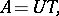whereis a partial isometric operator andis a positive operator. Any closed operatorhas a polar decomposition, moreover,(which is often denoted by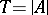), andmaps the closure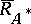of the domain of the self-adjoint operatorinto the closure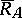of the range of(the von Neumann theorem, see ). A polar decomposition becomes unique if the source and target subspaces ofare required to coincide withand, respectively. On the other hand,can be always chosen unitary, isometric or co-isometric, depending on the relation between the codimensions of the subspacesand. In particular, ifthencan be chosen unitary and there is a Hermitian operatorsuch that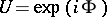. Then the polar decomposition oftakes the form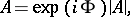entirely analogous to the polar decomposition of a complex number. Commutativity of the terms in a polar decomposition takes place if and only if the operator is normal (cf. Normal operator).

An expression analogous to the polar decomposition has been obtained for operators on a space with an indefinite metric (see , ).

A polar decomposition of a functional on a von Neumann algebrais a representation of a normal functionalonin the form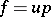, whereis a positive normal functional on,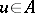is a partial isometry (i.e.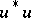and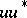are projectors), and multiplication is understood as the action onof the operator which is adjoint to left multiplication byin: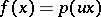for all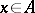. A polar decomposition can always be realized so that the condition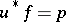is fulfilled. Under this condition a polar decomposition is unique.

Any bounded linear functionalon an arbitrary-algebracan be considered as a normal functional on the universal enveloping von Neumann algebra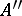; the corresponding polar decompositionis called the enveloping polar decomposition of the functional. The restriction of the functionaltois called the absolute value ofand is denoted by; the following properties determine the functional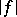uniquely: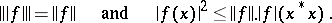In the case when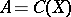is the algebra of all continuous functions on a compactum, the absolute value of a functional corresponds to the total variation of the measure determined by it (cf. also Total variation of a function).

In many cases a polar decomposition of a functional allows one to reduce studies of functionals on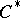-algebras to studies of positive functionals. It enables one, for example, to construct for each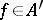a representationof the algebraon whichhas a vector realization (i.e. there are vectors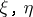insuch that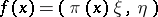,). The representationconstructed from the positive functionalusing the GNS-construction (of Gel'fand–Naimark–Segal) has that property.

The polar decomposition of an element of a-algebra is a representation of the element as the product of a positive element and a partial isometric element. Polar decomposition is not valid for all elements: in the usual polar decomposition of an operatoron a Hilbert space the positive term belongs to the-algebra generated by, but for the partial isometric term one can only state that it belongs to the von Neumann algebra generated by. That is why one defines and uses the so-called enveloping polar decomposition of an element: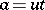, where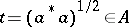andis a partial isometric element in the universal enveloping von Neumann algebra(it is assumed thatis canonically imbedded in).

How to Cite This Entry:
Polar decomposition. Encyclopedia of Mathematics. URL: http://encyclopediaofmath.org/index.php?title=Polar_decomposition&oldid=16949
This article was adapted from an original article by A.L. Onishchik, V.S. Shul'man (originator), which appeared in Encyclopedia of Mathematics - ISBN 1402006098. See original article